Editor’s Note: March 25-31, 2012 is VLOOKUP Week across the web. CFO is participating by offering a VLOOKUP Webcast on March 29, a VLOOKUP “Spicy Scale” formula edition of the CFO Learning Pro newsletter, and this VLOOKUP-themed article.

The VLOOKUP function is ubiquitous across spreadsheets in finance and accounting. One common problem is new values that get added to a lookup table. For example, say that you have 10,000 formulas that do a simple lookup, like this:

=VLOOKUP(A2,\$X\$1:\$Z\$7,3,False)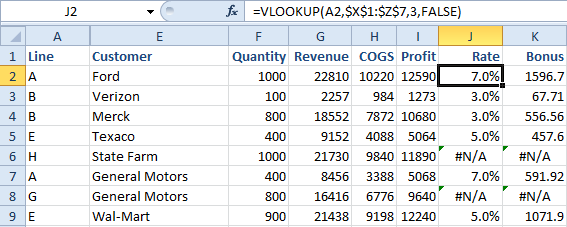Fig. 1 – The original lookup table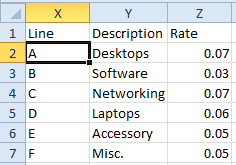Fig. 2 – A Simple VLOOKUP formula, Lines G & H are missing from the table.

If you need to add new rows to the lookup table, the obvious place is to type the new rows in X8:Z9. However, these rows are outside of the lookup table, so you must re-enter the existing VLOOKUP formulas.

Workarounds Before Excel 2007
Starting in Excel 2007, there is an amazing new solution to this problem. Before we get to the new solution, let’s cover the common workarounds that people have used for years.

• Use a named range such as ProdTable for X2:Z7. Use the named range in the formula: =VLOOKUP(A2,ProdTable,3,False). When you add new rows to the table, you change the definition once in the Name Manager and there is no need to edit the formulas.
• Refer to the entire column as the lookup table: =VLOOKUP(A2,\$X:\$Z,3,False).
• Excel is now smart enough to not include 1,048,569 blank rows in the formula
• Add the new rows to the middle of the table. Select the range X7:Z8. Type Alt+I followed by E, D, Enter. This will insert two new rows above row 7. The formulas will automatically be changed to include the range X2:Z9.

Ctrl+T: It Was Never Just for Formatting
Excel 2007 introduced a large icon called Format as Table. When you click on this icon, you are given 48 formats from which to choose. This might lead you to believe that the Format as Table command is all about making a table look nice. That is not the real benefit of the Format as Table command. In fact, when I need Table functionality, I use Ctrl+T which makes the range a table and applied default formatting.

The important functionality of a table is that pivot tables, charts, and VLOOKUP formulas that point to the table will automatically grow as you add new rows to the bottom of the table.  To do that, follow these steps:
1.  Start with a table as shown in Figure 1 above.
2.  Make sure that your VLOOKUP formula points to the entire table, including the headings. In this case, the lookup table would be \$X\$1:\$Z\$7.
3.  Select one cell inside the lookup table and press Ctrl+T.
4.  Excel will display the Create Table dialog shown in Figure 3. Click OK.
5   Excel will format the table as shown in Figure 4. While this is nice, it is not the important benefit of tables.
6.  Go to cell X8. Type two new rows below the table. Excel extends the table formatting to include the new rows. (Figure 5)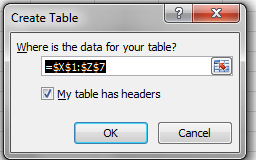Fig. 3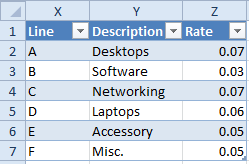Fig 4.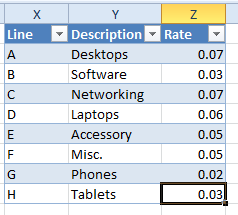Fig. 5

As soon as you finish entering G in cell X8, the #N/A error in cell J8 of Figure 2 has resolved itself. When you type the H in cell X9, the #N/A error in J6 has resolved itself. If you go back to cell J2 to look at the formula, something strange has happened. Excel automatically rewrote the formula to change the lookup range to point to \$X\$1:\$Z\$9.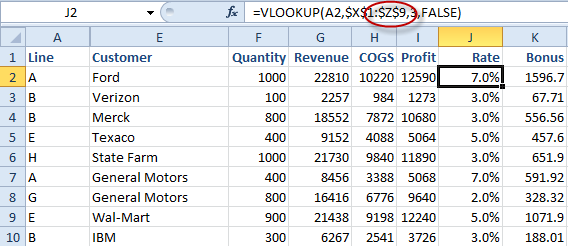Figure 6 – Excel automatically changed the lookup table argument in this formula.

This means that you can retroactively use Ctrl+T to change your existing lookup tables to a defined Table and Excel will handle rewriting the formula as you add new items to the bottom.

Bill Jelen is an Excel MVP. He will be conducting the 75-minute VLOOKUP webcast on March 29. Register at CFO.com.

### 2 responses to “Handle Growing Lookup Tables with a Two-Key Combo”

1.Patrick Coosemans says:

Dear,
I would like to subscribe at your weekly Excel newsletter, but received a error message when clicking on the hyperlink on your internet site : http://www.cfo.com/spreadsheets/.
Can someone help to subscribe
additional question : is this newsletter free? If not, what is the yearly fee?
thanks in advance

•Vincent Ryan says:

Sorry to inform, you, Patrick, but the Excel newsletter is no longer published. Occasionally, we publish stories that ran in the newsletter on CFO.com. Thank you for your interest.